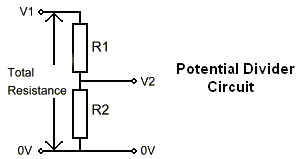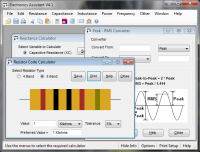# Potential Divider Calculator

These calculators perform calculations for potential divider circuits. The first one calculates the voltage from known resistor values, the second finds suitable resistor values if the desired output voltage is known. The third calculates R2 given V1, V2 and R1. Preferred resistor values are also calculated for the second and third calculators. Use the radio buttons on each calculator to select the preferred value series to use.#### Calculate Voltage Out (V2) from Resistors (R1 & R2):

 Enter R1: Ohms Kilohms Megohms Enter R2: Ohms Kilohms Megohms Enter V1: Volts Millivolts Microvolts V2 = ?

#### Calculate Resistors (R1 & R2) from Voltage Out (V2):

 Enter V1: Volts Millivolts Microvolts Enter V2: Volts Millivolts Microvolts Enter Total Resistance (R1 + R2): Ohms Kilohms Megohms Select preferred series (optional): E12 E24 E48 E96 E192 R1 = ? R2 = ? Preferred R1 = ? Preferred R2 = ?

#### Calculate Resistor  R1  from Voltage Out (V2) and R2:

 Enter V1: Volts Millivolts Microvolts Enter V2: Volts Millivolts Microvolts Enter R2: Ohms Kilohms Megohms Select preferred series (optional): E12 E24; E48 E96 E192 R1 = ? Preferred R1 = ?

#### Formula:

The voltage out of the divider is determined by the resistor values using the equation:

V2 = (R2 / (R1 + R2)) * V1

For further details about the operation of a potential divider see the technical data section here.

# If you found this calulator useful you should try Electronics Assistant!• All the online calculators and more in a stand-alone application
• Converts Resistor & Inductor colour codes, calculates LED series resistors, capacitance units, series / parallel resistors & capacitors, frequency, reactance & more
• Calculation of nearest preferred resistor values with a choice of 5 series from E12 to E192
• Print & save calculation results